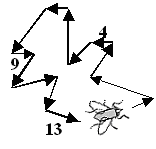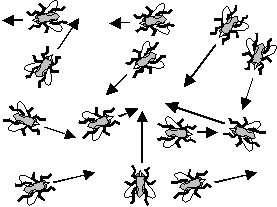# Averaging VectorsLet's look at bit closer at the averages one can take by considering a (localized) swarm of summer flies "circling" around like crazy, so that the ensemble looks like a small cloud of smoke. Check, maybe this Basic module first, if what follows seems a bit advanced."Localized" means that the swarm maintains a defined form (on average), so all flies are always inside this defined volume in space.In the case of the charge carriers in a piece of metal, it simple implies that the carriers stay inside the piece of metal.First we notice again that while the individual fly moves around quite fast, its average vector velocity <vi>t, averaged over time t, must be zero as long as the swarm as an ensemble doesn't move.In other words, the flies, on average, move just as often to the left as to the right etc. The net current flowing through some surface produced by all flies at any given instance, or by one individual fly after sufficient time is obviously zero for any reference surface you care to chose. This is illustrated schematically below.On the left hand picture 13 velocity vectors of an individual fly are shown; the chain of vectors closes so <vi>t = 0.On the right hand picture the same 13 velocity vectors are assigned to 1 fly each to demonstrate that the ensemble average treated below yields the same result, i.e. <ve> = 0, provided that each and every fly does the same thing on average.The average of the magnitude of the velocity of an individual fly, <|vi|>t = <vi>t, however, is obviously not zero - the fly, after all, is buzzing around at high (average) speed. Note the details in the equation above: Only the underlining of v is different!If we define <vi>t as follows, we have a simple way of obtaining the average of the magnitude (we take only the positive root, of course) .

<vi>t  =  + <(v2i)1/2>tv2 is a scalar, and the (positive) square root of v2 gives always the (positive) magnitude of v; i.e. |v|This is an elegant and workable definition, but beware:
<(v2)1/2> is not the same as
(<v2>)1/2!
Lets try it with a few arbitrary numbers      Þ

|v| = 3 4 6
<(v2)1/2> = <(9 + 16 + 36)1/2> = (61)1/2/3 = 4,47
(<v2>)1/2 = [(9 + 16 + 36)/3]1/2 = 20,331/2 = 4,51If we have <v>t = <(v2)1/2>t , we may also calculate the average (over time) of the velocity components in x, y, and z -direction, <vx>t , <vy>t , <vz>t , of an individual fly for a truly random movement. (We drop the index "i" now to make life easier).Again, the vector averages <vx> and so on of the vector components must be = 0 because in a truly random movement the components in + x and - x direction and so on must cancel on average.Since the magnitude |A|of a vector A is given by the square root of the scalar product of the vector with itself . We have
A · A  =  Ax · Ax + Ay · By + Az · Az = A2

A  =  |A|  =  (A2)½Since
<v2>t  =  <vx2>t + <vy2>t + <vz2>t   ,and since in a truly random movement we have
<vx>t  =  <vy>t = <vz>t   ,we end up with
<v2>t  =  3 <vx2>

<vx2>t  =  = 1/3 <v2>    .From this we finally get
<vx>t  =  <(vx2)½>t  =  (1/3)½  · <(v2)½>t  =  <v>t
3½In real life, however, the fly swarm "cloud" often moves slowly around - it has a finite drift velocity vD.
vD  =  <vi>tIn consequence, <vi>t is not zero, and <vi, +x>t (= average velocity component in +x direction) in general is different from <vi, –x>t.Note that the drift velocity by definition is an average over vectors; we do not use the < > brackets to signify that anymore. Note also, that the drift velocity of the fly swarm and the drift velocity of an individual fly must be identical if the swarm is to stay together.Without prove, it is evident that vD, i, x = <vi ,+x>t - <vi, –x>t and so on. In words: The magnitude of the component of the average drift velocity of fly number i in x-direction is given by the difference of the average velocity components in +x and x direction.This induces us to look now at the ensemble, the swarm of flies. What can we learn about the averages taken for the ensemble from the known averages of individual flies?As long as every fly does - on average - the same thing, the vector average over time of the ensemble is identical to that of an individual fly - if we sum up a few thousand vectors for one fly, or or a few million for lots of flies does not make any difference. However, we also may obtain this average in a different way:We do not average one fly in time obtaining <vi>t , but at any given time all flies in space.This means, we just add up the velocity vectors of all flies at some moment in time and obtain <ve>r , the ensemble average. It is evident (but not easy to prove for general cases) that
<vi>t  =  <ve>i.e. time average = ensemble average. The new subscripts "e" and "r" denote ensemble and space, respectively. This is a simple version of a very far reaching concept in stochastic physics known under the catch word "ergodic hypothesis".This means that in "normal" cases, it doesn't matter how averages are taken. This is the reason why text books are often a bit unspecific at this point: It is intuitively clear what a drift velocity is and we don't have to worry about how it is obtained. It also allows us to drop all indices from now on whenever they are not really needed.In our fly swarm example, the drift velocity <vD> = <vi> is usually much smaller than the average <vi> of the velocity magnitudes of an individual fly.The magnitude of <vD> is the difference of two large numbers - the average velocity of the individual flies in the drift direction minus the average velocity of the individual flies in the direction opposite to the drift direction.This induces an asymmetry: From a knowledge of the drift velocity only, no inference whatsoever can be made with regard to <vi, +x> , <vi, –x> or <vi> whereas knowlegde of <vi, +x> and <vi, –x> tells us all there is to know in x-directionThis teaches us a few things:1. Don't confuse <v> with <v>. The first quantity - for our flies - is zero or small, whereas the second quantity is large; they are totally different "animals".2. This means in other words: Don't confuse the property of the ensemble - the drift velocity vD of the ensemble or swarm - with the properties of the individuals making up the ensemble.2.1.1 Ohms Law and Materials Properties2.1.2 Ohms Law and Classical PhysicsTemperatur, Gleichverteilungssatz etc. - die GrundlagenSolution to Exercise 2.1-1: Derive and discuss numbers for µ and vD

© H. Föll (MaWi 2 Skript)